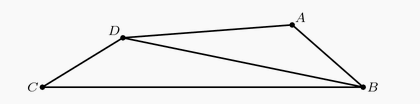###### back to index | new

Two of the three sides of a triangle are 20 and 15. Which of the following numbers is not a possible perimeter of the triangle?

In $\triangle BAC$, $\angle BAC=40^\circ$, $AB=10$, and $AC=6$. Points $D$ and $E$ lie on $\overline{AB}$ and $\overline{AC}$ respectively. What is the minimum possible value of $BE+DE+CD$?

Real numbers $a$ and $b$ are chosen with $1 < a < b$ such that no triangle with positive area has side lengths $1$, $a$, and $b$ or $\frac{1}{b}$, $\frac{1}{a}$, and $1$. What is the smallest possible value of $b$?

Three distinct segments are chosen at random among the segments whose end-points are the vertices of a regular 12-gon. What is the probability that the lengths of these three segments are the three side lengths of a triangle with positive area?

In quadrilateral $ABCD$, $AB = 5$, $BC = 17$, $CD = 5$, $DA = 9$, and $BD$ is an integer. What is $BD$?In a triangle with integer side lengths, one side is three times as long as a second side, and the length of the third side is 15. What is the greatest possible perimeter of the triangle?

Shaina has one stick of length $a$ cm and another of length $b$ cm, where $a \ne b$. She needs a third stick with length strictly between 8 cm and 26 cm to make the third side of a triangle. What is the product $ab$?

Let positive real number $x$, $y$, and $z$ satisfy $x+y+z=1$. Find the minimal value of $u=\sqrt{x^2 + y^2 + xy} + \sqrt{y^2 +z^2 +yz} +\sqrt{z^2 +x^2 + xz}$

How many distinct isosceles triangles having sides of integral lengths and perimeter 113 are possible?